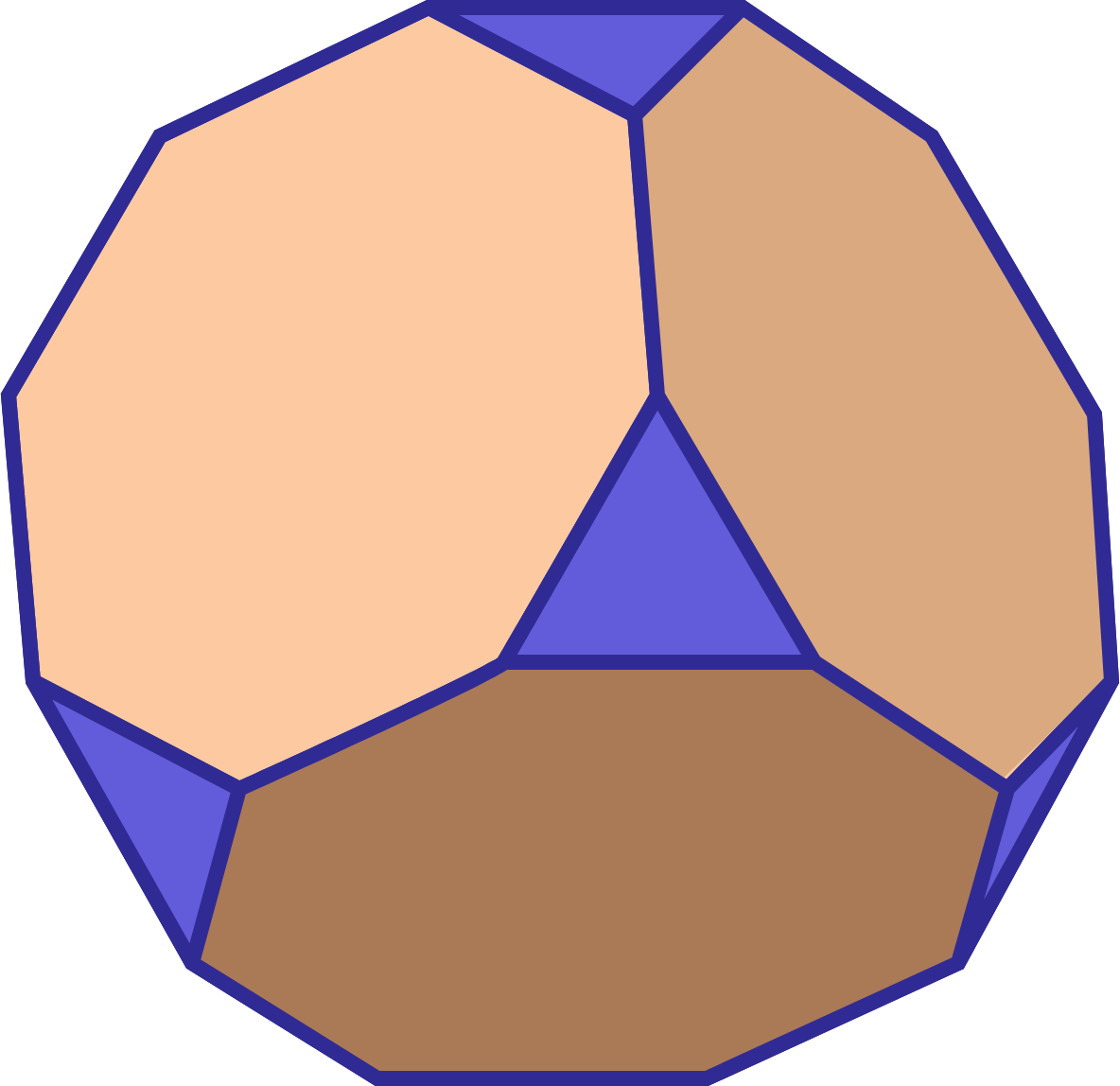Geometry Level 3

The artistic carpenter forms a tetradecahedron from a cube with side length 3". For each of the cube's vertices, he measures an inch out on the edges, and cuts out the resulting right tetrahedron from each corner, leaving 6 octagonal faces, and 8 triangular faces. The volume is $\frac{a}{b}$ where $a$ and $b$ are relatively prime positive integers and the surface area is $c+d\sqrt{e}$, and $e$ is prime. What is the sum $a+b+c+d+e$?×